# Iodine dichloride (ICl2-) lewis dot structure, molecular geometry, polar or non-polar, hybridization

Home  > Chemistry Article > ICl2- lewis structure and its molecular geometryIn this tutorial, we will discuss ICl2- lewis structure, molecular geometry, polar or nonpolar, hybridization, etc.

 Name of Molecule Iodine dichloride Chemical formula ICl2- Molecular geometry of ICl2- Linear Electron geometry of ICl2- Trigonal bipyramidal Hybridization Sp³d Nature Nonpolar molecule Bond angle 180° Total Valence electron in ICl2- lewis structure 22
Page Contents

## How to draw ICl2- lewis structure?

ICl2- lewis structure contains one iodine atom at the middle position whereas two chlorine atoms at the surrounding position. There are three lone pairs present on the central atom of ICl2- lewis structure.

Also, the iodine central atom in ICl2- lewis structure violates the octet as it is holding more than 8 electrons in its octet shell.

## Follow some steps for drawing the lewis structure of ICl2-

1. Count total valence electron in ICl2-

As the lewis diagram is all about filling the valence electron around the atoms within a molecule, hence, find the total valence electron in ICl2- molecule.

To count the valence electron in ICl2- molecule, look at the periodic group of iodine and chlorine atom in the periodic table. Since both iodine and chlorine atom belongs to the same periodic group(Group 17), they have same valence electrons.

⇒ Total number of the valence electrons in chlorine atom = 7

⇒ Total number of the valence electrons in iodine atom = 7

∴ Total number of valence electron available for ICl2- lewis structure = 7 + 7(2) + 1 = 22 valence electrons         [∴ICl2- molecule has one iodine and two chlorine atom with one negative charge ion]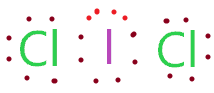2. Find the least electronegative atom and placed it at center

A less electronegative atom is capable to share more electrons, hence, the least electronegative atom takes the middle position in the lewis diagram.

In the case of the ICl2- molecule, both iodine and chlorine atoms belong to the same periodic group but “the electronegativity of an element decrease as we down the group”.

Hence, the iodine atom(2.66) is less electronegative than the chlorine atom(3.16), so, place iodine at the central position and chlorine spaced evenly around it.3. Connect outer atoms to central atom with a single bond

In this step, connect both chlorine atoms to the iodine central atom with the help of a single bond.Now count the valence electrons we used in the above structure. One single bond means two bonded pairs of electrons. In the above structure, we use two single bonds for connecting both side chlorine atoms to the iodine central atom.

Therefore, (2 single bond × 2) = 4 valence electrons we used in the above structure from a total of 22 valence electrons that is available for ICl2-.

∴ (22 – 4) = 18 valence electrons

So, we are left with 18 valence electrons more.

4. Place remaining valence electrons starting from outer atom first

Now start placing the remaining valence electrons on the outer atom(chlorine) to complete their octet i.e. providing 8 valence electrons in the outer shell of each chlorine atom.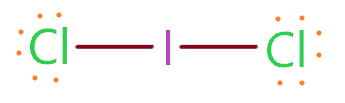As you see in the above figure, we have place 6 valence electrons represented as dots around both sides chlorine atoms. This is because each chlorine atom already sharing two valence electrons with the help of a single bond.

Hence, (one single bond + 6 valence electron as dots) means a total of 8 valence electrons are present around each chlorine atom, hence, both of them completed their octet comfortably.

Now count the remaining valence electrons. In the above structure, (12 valence electrons represented as dots + 2 single bond that contains 4 electrons) are uses, hence, (12 + 4) = 16 valence electrons are used from total of 22 valence electron available for ICl2-.

∴ (22 – 16) = 6 valence electrons

So, we are left with only 6 valence electrons.

5. Complete central atom octet and make covalent bond if necessary

We have 6 remaining valence electrons and iodine is the central atom that needs 8 electrons in its octet to attains stability. But the iodine atom already has 4 electrons in its octet because of two single bonds connected to it.

But iodine atom can expand the octet i.e. having more than 8 valence electrons in the outer shell, this is because iodine is below period two on the periodic table and have an extra d-orbital available hence, it has the ability to expand the octet.

So, put these 6 remaining valence electrons around the iodine central atom.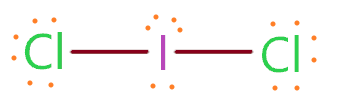As we see in the above figure, the iodine central atom gets 10 electrons(6 represented as dot + 2 single bonds) in its outer shell and violates the octet rule. But this is not the issue, some elements can violate the octet to gains stability.

So, both atoms (iodine and chlorine) completed their octet and we use all the total valence electrons that are available for drawing the ICl2- lewis structure.

Now we will check the stability of the above structure with the help of a formal charge concept.

6. Check the stability with the help of a formal charge concept

To calculate the formal charge on an atom. Use the formula given below-

⇒ Formal charge = (valence electrons – lone pair electrons –  1/2 bonded pair electrons)

First, count the formal charge on the chlorine atom.

For chlorine atom:

⇒ Valence electrons of chlorine = 7

⇒ Lone pair electrons on chlorine = 6

⇒ Shared pair electrons around chlorine(1 single bond) = 2

∴ (7 – 6 – 2/2) = 0 formal charge on both sides chlorine atom.

For iodine atom

⇒ Valence electrons of iodine = 7

⇒ Lone pair electrons on iodine = 6

⇒ Shared pair electrons around iodine (2 single bonds) = 4

∴ (7 – 6 – 4/2) = -1 formal charge on the iodine central atom.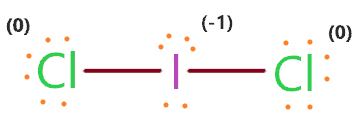The overall formal charge in ICl2- lewis structure is -1 which is equal to the charge on the ion(ICl2- molecule has one negative charged ion).

So, the above structure is the most stable, and lastly, put the bracket around both sides of the Iodine dichloride lewis structure and show its negative charged ion.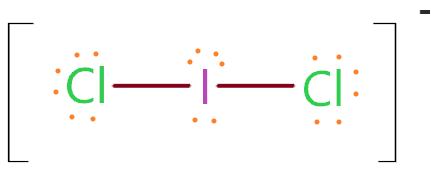### ICl2- lewis structure

The lewis structure for ICl2+For ICl2+, we have 20 valence electrons and the central atom iodine has 2 lone pairs and 2 shared pairs.

## What is the molecular geometry of ICl2-?

The molecular geometry of ICl2- is linear and the electron geometry is trigonal bipyramidal, this is because the iodine central atom has three lone pairs on an equatorial position and 2 bonded pairs are attached to it.

As per VSEPR theory, the three lone pairs on iodine central atom repel each other as well as adjacent bonded pairs, as a result, the bond pair and lone pair pushed apart from each other and distorted the shape.

∴ The resultant molecular shape of ICl2- appears linear.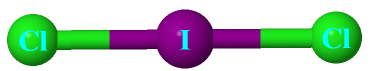We can also find the molecular and electron geometry of ICl2- with the AXE method.

AXE is the simple generic formula that is based on bonded atom and lone pair on central atom of a molecule.

AXE notation for ICl2- molecule:

• A denotes the central atom, so, iodine is the central atom, A = Iodine
• X represent the bonded atoms to the central atom, as we know, iodine is bonded with two chlorine atoms. Therefore, X = 2
• E represent the lone pair on the central atom, as per ICl2- lewis structure, iodine has three lone pairs. Hence, E = 3

So, the AXE generic formula for the ICl2- molecule becomes AX2E3.Image source

VSEPR theory or chart says, if the central atom of any molecule is attached with two bonded atoms and contains a total of three lone pairs then the molecular shape of that molecule is linear, and electron geometry is trigonal bipyramidal in nature.## Hybridization of ICl2-

To find the hybridization of ICl2-, just determine the steric number of the central atom.

The steric number can be found by the addition of lone pair and bonded atom to a central atom.

∴ Steric number of ICl2- = (Number of bonded atoms to iodine + Lone pair on iodine central atom)

The iodine central atom is bonded with two chlorine atoms and contains a total of three lone pairs according to the ICl2- lewis structure.

∴ Steric number of ICl2- = (2 + 3) = 5

 Steric number Hybridization 1 S 2 Sp 3 Sp² 4 Sp³ 5 Sp³d 6 Sp³d²

So, we get the Sp3d hybridization for ICl2- molecule for a steric number of five.

## Iodine dichloride polarity: is ICl2- polar or nonpolar?

A polar molecule has non zero dipole moment and charges are distributed asymmetrical all over the atoms. In nonpolar molecules, charges are the same, hence, all dipole is canceled out.

So, Is ICl2- polar or nonpolar? ICl2- the molecule is nonpolar in nature because its molecular shape is linear that means dipole that is induced because of the difference in electronegativity value of iodine and chlorine atoms are strictly opposite in direction to each other.

“Also, the three lone pairs of electrons on iodine central atom are 120 away from each adjacent one, a rotationally-symmetric configuration; so, the lone-pair-bonding-pair repulsions sum to cancel out as well.”[check more here]

The dipoles that are generated along with the bonds(I-Cl) will cancel out to give a net dipole moment of zero in all directions irrespective of the difference in electronegativity value between iodine and chlorine.

Hence, the cancellation of dipole and symmetric geometry makes the ICl2- nonpolar in nature.

## FAQ

### What makes the molecular geometry of ICl2- linear?

As per the ICl2- lewis structure, iodine is the central atom that contains 3 lone pairs and 2 bonded pairs attached to it. These electron pairs (lone pair + bond pair) will repel each other and tried to go far away to minimize the repulsion.

Hence, in all this process, these electron pairs around iodine atom take the position where repulsion between them remains minimum and the final molecular shape of ICl2- appears as linear geometry with bond angle 180°.

Also, the generic formula for ICl2- is AX2E3 which implies the linear molecular shape and trigonal bipyramidal electron geometry.

### Why does the iodine central atom in ICl2- lewis structure violate the octet and attains more than 8 electrons?

This is because the iodine atom is below period two on the periodic table and has an access to the d-orbital, hence when it becomes necessary, the iodine atom can open its extra d-orbital and attains more than 8 electrons to complete the octet.

Examples of some elements that can expand their octet whenever it’s required – Sulfur, phosphorus, silicon, and chlorine, etc.

“Elements in the first 2 periods of the Periodic Table do not have access to the d sublevel and must adhere to the octet rule.”

## Summary

• The total valence electron is available iodine dichloride lewis structure is 22.
• The steric number of iodine central atom in the ICl2- the molecule is 5, thus, it forms Sp3d hybridization.
• The nature of ICl2- is nonpolar because all dipole that generated along the bond will cancel out because of symmetrical geometry of it.
• A total of 9 lone pairs(3 lone pairs on central atom whereas 6 lone pairs on outer atoms) and 2 bonded pairs are present in ICl2- lewis structure.
• The molecular geometry of ICl2- is linear whereas electron geometry is trigonal bipyramidal.
• The bond angle in ICl2- molecule is 180º.
##### Subscribe to Blog via Email

Join 2 other subscribers

Share it...